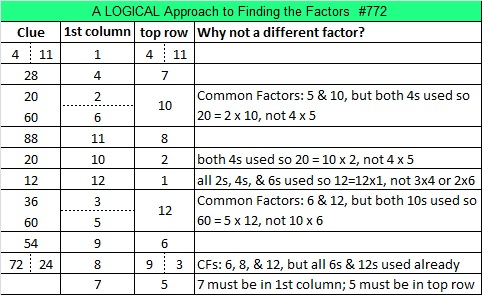# 772 and Level 5

• 772 is a composite number.
• Prime factorization: 772 = 2 x 2 x 193, which can be written 772 = (2^2) x 193
• The exponents in the prime factorization are 2 and 1. Adding one to each and multiplying we get (2 + 1)(1 + 1) = 3 x 2  = 6. Therefore 772 has exactly 6 factors.
• Factors of 772: 1, 2, 4, 193, 386, 772
• Factor pairs: 772 = 1 x 772, 2 x 386, or 4 x 193
• Taking the factor pair with the largest square number factor, we get √772 = (√4)(√193) = 2√193 ≈ 27.78488798.Here is a factoring puzzle to try:Print the puzzles or type the solution on this excel file: 12 Factors 2016-02-25

————————————-

Here’s more about the number 772:

24² + 14² = 772 so 772 is the hypotenuse of a Pythagorean triple, and 380² + 672² = 772². 380-672-772 is calculated from 24² – 14², 2(24)(14) , 24² + 14².

22² + 12² + 12² = 772, making 772 the sum of three square numbers.

772 is also the sum of two consecutive prime numbers: 383 + 379 = 772.

OEIS.org informs us that 772 is the smallest number that is the sum of three triangular numbers 21 different ways. I decided to find all those ways for myself and share them here. (If zero wasn’t named the zeroth triangular number, there would “only” be 20 ways.)————————————-## One thought on “772 and Level 5”

1.theintrovertman

Marvellous analysis, love you.👌👌👌👏👏👏👏👏👏🙌🙌🙌🙌🙌🙌😘😘🌷🌷🌷💝💝💝

This site uses Akismet to reduce spam. Learn how your comment data is processed.